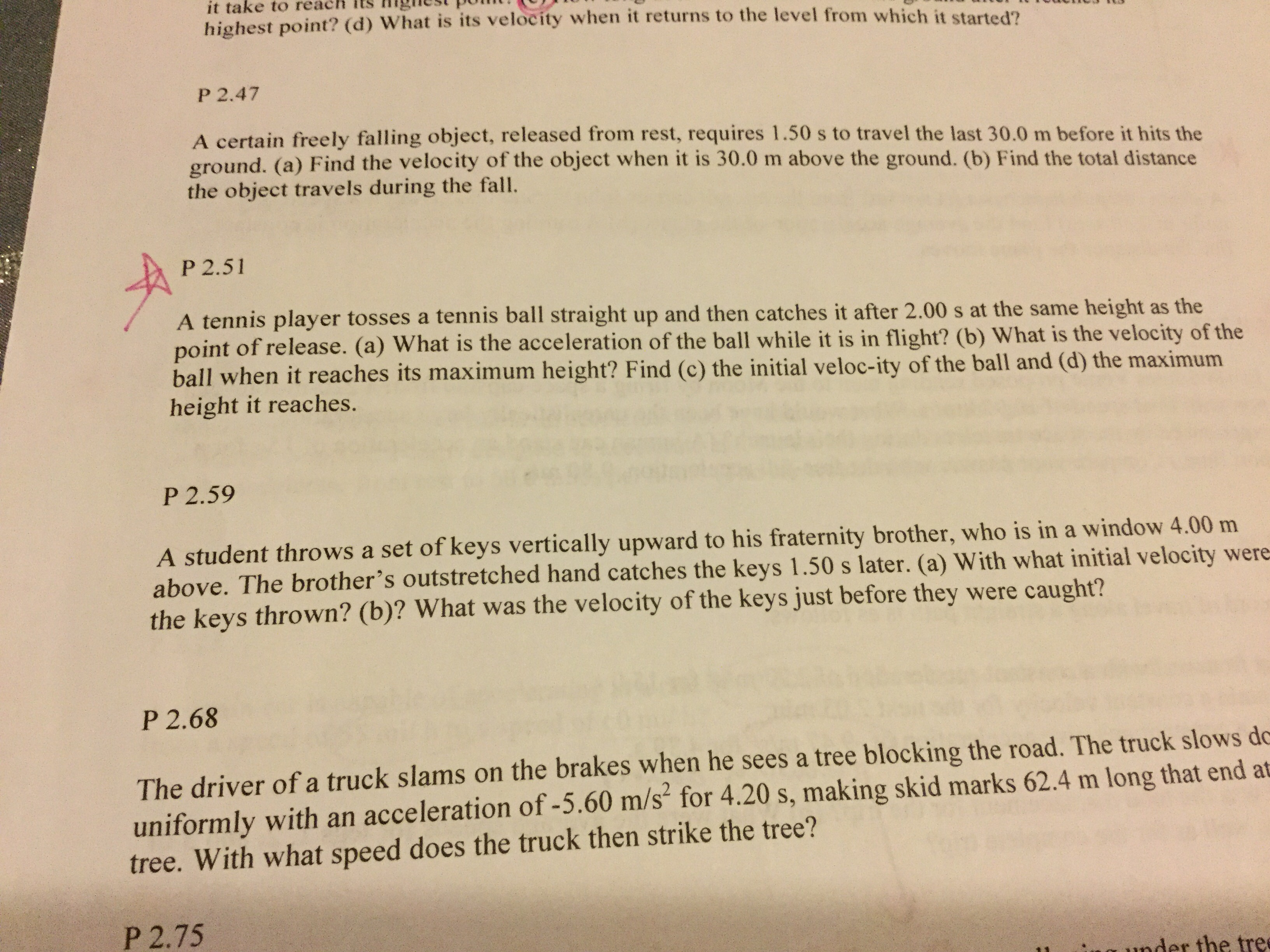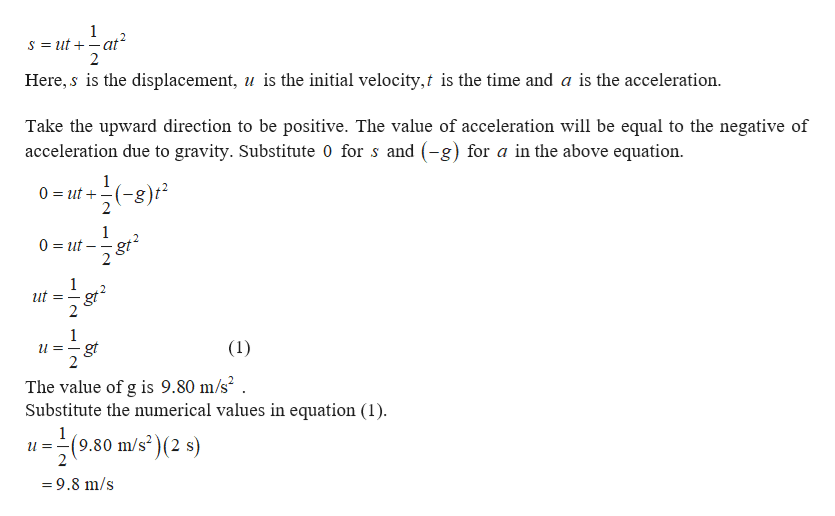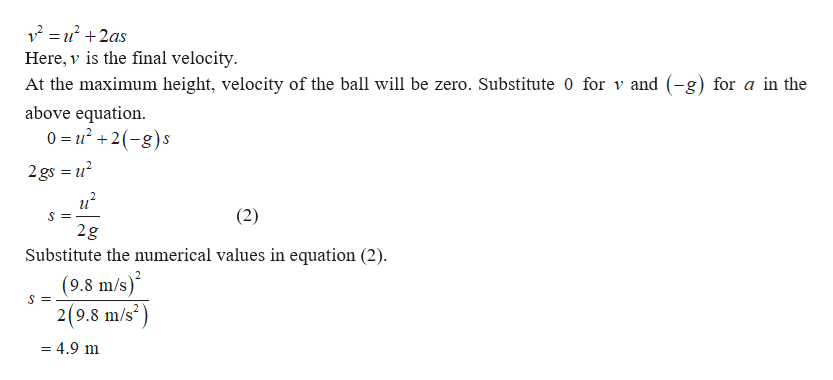it take to reachhighest point? (d) What is its velocity when it returns to the level from which it started?P 2.47A certain freely falling object, released from rest, requires 1.50 s to travel the last 30.0 m before it hits theground. (a) Find the velocity of the object when it is 30.0 m above the ground. (b) Find the total distancethe object travels during the fallP 2.51A tennis player tosses a tennis ball straight up and then catches it after 2.00 s at the same height as thepoint of release. (a) What is the acceleration of the ball while it is in flight? (b) What is the velocity of theball when it reaches its maximum height? Find (c) the initial veloc-ity of the ball and (d) the maximumheight it reaches.P 2.59A student throws a set of keys vertically upward to his fraternity brother, who is in a window 4.00 mabove. The brother's outstretched hand catches the keys 1.50 s later. (a) With what initial velocity werethe keys thrown? (b)? What was the velocity of the keys just before they were caught?P 2.68The driver ofa truck slams on the brakes when he sees a tree blocking the road. The truck slows dcuniformly with an acceleration of -5.60 m/s for 4.20 s, making skid marks 62.4 m long that end attree. With what speed does the truck then strike the tree?P 2.751nder the tre

Question

On question p 2.51, I am confused on part c and dhelp_outlineImage Transcriptioncloseit take to reach highest point? (d) What is its velocity when it returns to the level from which it started? P 2.47 A certain freely falling object, released from rest, requires 1.50 s to travel the last 30.0 m before it hits the ground. (a) Find the velocity of the object when it is 30.0 m above the ground. (b) Find the total distance the object travels during the fall P 2.51 A tennis player tosses a tennis ball straight up and then catches it after 2.00 s at the same height as the point of release. (a) What is the acceleration of the ball while it is in flight? (b) What is the velocity of the ball when it reaches its maximum height? Find (c) the initial veloc-ity of the ball and (d) the maximum height it reaches. P 2.59 A student throws a set of keys vertically upward to his fraternity brother, who is in a window 4.00 m above. The brother's outstretched hand catches the keys 1.50 s later. (a) With what initial velocity were the keys thrown? (b)? What was the velocity of the keys just before they were caught? P 2.68 The driver ofa truck slams on the brakes when he sees a tree blocking the road. The truck slows dc uniformly with an acceleration of -5.60 m/s for 4.20 s, making skid marks 62.4 m long that end at tree. With what speed does the truck then strike the tree? P 2.75 1nder the tre fullscreen
Step 1

(c)Consider the motion of the ball from its release to the time when it caught. It is given that the ball is caught at the same height as the point of release. This implies the net displacement of the ball is zero. The equation of motion is given byhelp_outlineImage Transcriptionclose1 s ut at 2 Here, s is the displacement, u is the initial velocity,t is the time and a is the acceleration Take the upward direction to be positive. The value of acceleration will be equal to the negative of acceleration due to gravity. Substitute 0 for s and (-g) for a in the above equation 1 0 ut ) 2 1 0 ut 2 1 ut gt 1 (1) gt 2 =_ The value of g is 9.80 m/s2 . Substitute the numerical values in equation (1) 1 (9.80 m/s2 )(2 s) =9.8 m/s fullscreen
Step 2

(d)The equation of motion that can be used to determ...help_outlineImage Transcriptionclose2u 2as Here, v is the final velocity At the maximum height, velocity of the ball will be zero. Substitute 0 for v and (-g) for a in the above equation. 0 = u2(-g)s S 2gs (2) S = 2g Substitute the numerical values in equation (2) (9.8 m/s) 2(9.8 m/s2 S = =4.9 m fullscreen

Want to see the full answer?

See Solution

Want to see this answer and more?

Our solutions are written by experts, many with advanced degrees, and available 24/7

See Solution
Tagged in

Newtons Laws of Motion